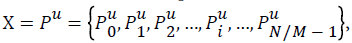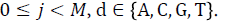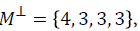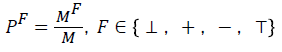###### Indexed In
• Open J Gate
• Genamics JournalSeek
• CiteFactor
• Cosmos IF
• Scimago
• Ulrich's Periodicals Directory
• Electronic Journals Library
• RefSeek
• Hamdard University
• EBSCO A-Z
• Directory of Abstract Indexing for Journals
• OCLC- WorldCat
• Proquest Summons
• Scholarsteer
• Virtual Library of Biology (vifabio)
• Publons
• Geneva Foundation for Medical Education and Research
###### Journal Flyer###### Open Access Journals

Research Article - (2016) Volume 8, Issue 6

# Variant Maps on Normal and Abnormal ECG Data Sequences

Yan Ji1, Jeffrey Zheng1,2*, Yinfu Xie3 and Tao Shou3
1School of Software, Yunnan University, Kunming 650091, China
2Key Lab of Yunnan Software Engineering, Kunming 650091, China
3The First People’s Hospital of Yunnan Province, Kunming 650031, China
*Corresponding Author: Jeffrey Zheng, School of Software, Yunnan University, Kunming 650091, China, Tel: +86-1310883909 Email:

## Abstract

ECG data sequences are classical and most reliable clinical data for patients to provide complex physiological and pathological information diagnosing various heart diseases. Extracting dynamic information from ECG signal time sequences, Poincare maps have developed as classical assistant tools using two dimension maps as important basis for medical doctors to diagnose multiple cardiovascular diseases. Since simulation systems of human heart could be extremely complicated on chaos behaviors, Poincare maps based on paired measures have some limitations to excavate ECG data sequences on special physiological and pathological information. In this paper, we propose a new measuring model based on multi-dimensional measurements using variant maps to handle ECG data sequences in refined visual representations. System architecture of this model and their core components are discussed. Under this construction, normal and abnormal ECG data sequences can be represented as variant maps. Sample results are illustrated as a set of two dimensional variant maps for selected ECG data sequences.

Keywords: Heart diseases, ECG data sequences, Variant maps, Visualization, Probability measurement

#### Introduction

Advanced health informatics is listed as one of the fourteen engineering grand challenges for the 21st century . Activities in this field include acquiring, managing, and using biomedical information. It is necessary to establish health assurance systems from personal to global levels, to enhance the quality and efficiency of medical care and the response to widespread public health emergencies .

Most people are well known that the Electrocardiogram (ECG) are represented as irregular curves on long grid papers to record electrical activities of a heart. However beside physician experts, a few people can really understand possible meanings of complicated ECG curves. From a measurement viewpoint, ECG signals are physical indices as heart activities in cycles to indicate various electrical signals. There are various states before contraction, pre excites on the heart, and then spreading to the patient’s body, identifying electrical levels on the body to produce potential differences. ECG devices record these signals in potential differences, such graphic patterns are called electrocardiogram .

Due to noninvasive nature and intrinsic information on heart activities , ECG signals are the most common signals of heart diseases widely used in general medical practice over the world of health environment. ECG can examine the nature of the diagnosis of patient’s heart with arrhythmia or rhythmia properties. The collection of ECG data obtained from volunteers is useful with huge amount of data sequences. In modern world, heart diseases are already occurring as the largest portion of daily health diseases on the transmission system in human society . The analysis of ECG data sequences plays an essential role to diagnose various heart diseases in clinic practices in the field of health information to be giant projects in advanced medical practices over the world.

At present, in the direction of medical diagnosis on ECG signals, a useful processing model is based on Poincare maps invented by the eminent French scientist in 100 years ago that are widely applied in modern chaos theory and complex systems. Based on a set of paired ECG data on selected sequences, this type of maps can be generated as visual maps in the identification of different types of cardiac arrhythmias . Using Poincare maps, ECG signals are transformed as scattered point diagram based on a nonlinear analysis method as the foundation. It is feasible for computer software to convert longer ECG data sequences into simple graphical representation as 2D maps .

Applying modern probability and statistical analysis tools, this type of processing models could be useful to make feature extractions mining implicit information in a large set of sample ECG data sequences. It is difficult for classical ECG technologies to identify a particular class of heart diseases with nonlinear physiological or pathological information. Due to specific types of diseases may be observed as a family of similar curves with the same variation on measurements from data sequences, their spectrum in feature maps may be identified as normal rhythm and arrhythmia ECG signals.

To explore efficient technology analysis ECG signals using advanced methodologies, a new model based on variant maps [2-10] are applied to ECG data sequences.

In consequent section, variant maps on ECG data sequences to identify normal/abnormal ECG data sequences will be described and different visual results will be analyzed.

#### System Architecture

In this section, architecture of a variant map system and its core components are discussed to process ECG data sequences generating variant maps with the use of diagrams. The refined definitions and equations of this system are described.

Architecture

The Architecture of the ECG Variant Map system is composed of three components: Transformation Component TC, Measure Component MC, and Visualization Component VC shown in Figure 1. Each component is composed of one ~ four modules respectively discussed in the next subsection.

A list of parameters can be described as follows:Under this construction, the component of TC processes a selected ECG data sequence E and two control parameters {W, R} as inputs: E an ECG data sequence with N elements, W pre-set Window-Size value, and R the steady state interval value. Undertaken relevant computations and processing, the output of TC component is composed of a Pseudo-DNA data sequence that will be provided as an input data sequence for the MC. Inputs from TC with a segment value M as a control parameter are processed in the MC. The output of the MC is organized as four vectors of probability measurements. Selecting two vectors from the four probability vectors, they become the input data of the VC component, the output of the VC is composed of a pair of position values created from each M values from the two selected vectors. The pair of positions in the VC determines a projected position as a visual point. After all elements on the selected ECG data sequence are processed, multiple segments are transformed as a set of multiple paired values to generate relevant graphs to indicate their distribution properties on 2D variant maps respectively.

Core modules

It is essential to describe core modules in each component. Three components are described as follows.

The TC component

The TC component is composed of four modules: Basic Value Computation Module BVCM, Range Value Computation Module RVCM, Value Computation Module VCM and Pseudo-DNA Generator Module PDGM shown in Figure 2. The output of the TC component provides its output as the input of the MC component.

Main I/O parameters of the TC component are organized in three groups (Input, Intermediate and Output):

Input group

E ECG signals with N elements;

W pre-set Window-Size value;

R the steady state interval value;

Intermediate output/input groupBasic value sequence;RV:Range value sequence;V:Value sequence;

Output group:Pseudo-DNA sequence.

The inputs of BVCM are E, W and the output is BV. The inputs of RVCM are E, W, R and the output is RV. The inputs of VCM are E, W, R, BV, RV and its output is V. This vector provides the input of the PDGM, and the PDGM’s output is PV to be transformed as a Pseudo- DNA sequence.

The MC component

The MC component is composed of three modules: Variant Measurement VM, Probability Measurement PM, and Selection Mechanism SM shown in Figure 3. The inputs of VM component are composed of a Pseudo-DNA sequence PV and a segment length M, its output is a sequence composed by four symbols:The input of the PM component is the output of VM component, and the output is composed of probability measurement sequences: four sets of probability measurements. The input of SM component is the four sets of probability measurements and the output is selected from two sets of probability measurementsThe MC component is shown as Figure 3 and its I/O parameters are listed as follows.

Input group: PV: Pseudo-DNA sequence composed by four bases: {A, C, G, T};

M: segment length;

Intermediate output/input group: N: length of ECG sequence;

0-1 sequence: sequences obtained by respectively map {A, C, G, T};

F: multiple sequences are composed by four symbol:Four possible measures:probability measure sequences of four symbols:Output group:probability measure sequences of two symbols:The inputs of MC component are a Pseudo-DNA sequence PV, M and intermediate input parameters. Final output is probability measure sequences of two symbols. In this paper, we select a paired of probability measure sequences on two symbols and respectively.

The VC component

This VC component is composed of one module only: Point Generator PG. The input of PG is a set of paired measures X and Y;Final output is 2D variant maps of selected ECG data sequences.

The VC component is shown in Figure 4 and its I/O parameters are listed as follows.

Input groupa set of paired probability measure sequences;

Output group: 2D map This part uses paired values to repeat process on rest segments generated from the selected ECG data sequence.

#### Detailed Description

Section 2 provides system description on ECG variant map system, it is necessary to make explanation for its further details.

Parameter descriptionSelected ECG data sequence with length N.D =Generated Pseudo-DNA sequence PV with lengthsequences with length N,0-1 sequence of every segmentand length of every segmentTransformation component

BVC module is used to compute the basic value of the selected ECG data sequence. Equation of computation BV is listed as follows:RVC module is used to compute the range value of the selected ECG data sequence. Equation of computation RV is listed as follows:maxMax value in Window-Size.Min value in Window-Size.

VC module is used to compute final value of the selected ECG data sequence. Equation of computation V is listed as follows:According to the following method, a Pseudo-DNA sequence is produced:Measurement component

Pseudo-DNA sequence is converted into four 0-1 sequences. Using the symbol to show Map A, Map G, Map C, Map T Detailed method [1-10] is described as follows:Example: A Pseudo-DNA sequence is AGCTAAAGGGTTCGCTACGCGGCTA. Then four distinct sequences can be generated:

MapA: XA=1000111000000000100000001;

MapG: XG=0100000111000100001011000;

MapC: XC=0010000000001010010100100;

MapT: XT=0001000000110001000000010.

Each 0-1 sequence can be segmented by length M into multiple segments and usingto show 0-1 sequence of the i-th segment.Example: If a 0-1 sequence ofis 0010000000001010010100100 and length M=5, then this sequence can be arranged as following segments:0-1 sequences are converted into variant sequences through method  as follows:0-1 sequences are converted into variant sequences of being composed variant symbolthrough VM component.Example: ifthenIf usingrepresent the number of variant symbol F of every segment variant sequence andthenThe equation of computation probability measures on each segmented variant sequence is listed as follows:Visualization component

Using this set of measurements, projective functions can be established to select a pair of values to transform an ECG data sequence into a 2D map as follows.

Letbe the i-th pair of values,andThen each pair of values locates a specific position on a 2D map for the selected ECG data sequence.

Eachdetermines a specific position on 2D plane. A series of projections are repeated on relevant segments and each segment corresponding to a projective point on the 2D map. This makes all processed measurements be their projective points, and finally to generate a 2D maps for the selected ECG data sequence. Each segment on the ECG data sequence generates a specific point on a 2D map; it is essential to recursively process all relevant segments using the VM.

#### Results of ECG Variant Maps

Applying this process based on variant model and visualization method, normal and abnormal ECG data sequences can be distinguished.

Sample ECG data information

In this paper, all ECG data sequences are provided by the First People’s Hospital of Yunnan Province. A total amount of ECG data has 500 MB to collect from 220 thousand records. All records are diagnosed and classified by ECG experts. Among this data set, normal ECG data has about 138 MB and abnormal ECG data has about 362 MB. The data format is briefly shown in Figure 5.

It is always difficult to imagine map results from control parameters directly. A list of controlled effects will be illustrated in this section. Let readers easier look at different visual effects via sample results of ECG variant maps under various controlled parameters.

Normal and abnormal ECG samples

Applying normal and abnormal ECG data sequences, different conditions illustrate their spatial distributions in a controllable environment. Sample 1D maps are shown in Figure 6.

ECG variant maps based on Pseudo-DNA

Four maps of on bases of Pseudo-DNA generated by normal and abnormal ECG data sequence are shown in Figure 7.

Figure 7: The ECG Variant Maps of ECG data sequences; (a-d) normal results on (a) Map A, (b) MapG, (c) Map C, (d) Map T; (e-h) abnormal results on (e) Map A, (f)Map G (g) Map C, (h) Map T respectively.

In Figure 7, eight ECG variant maps of ECG data sequences are listed under the condition M=79, N=25561, W=30, R=0.95. Where maps (a-d) are shown the results of normal ECG sample on Map A, Map G, Map C, Map T respectively. Maps (e-h) are shown the results of abnormal ECG sample on Map A, Map G, Map C, Map T respectively.

Sample results of ECG variant maps on different parameters

In Figure 8, six 2D maps are illustrated in the range of M=70-100, N=25561, R=0.95 for comparison, its scatter points are more compact with M value. Scatter points are mostly distributed between 0.2 to 0.6 region on X-axis.

Figure 8: Normal ECG variant maps on six M values; (a-f) M= {70, 76, 82, 88, 94, 100}; N=25561, W=30, R=0.95.

In Figure 9, six 2D maps are illustrated in the range of M=70-100, N=25561, R=0.95 for comparison, scatter points illustrate arc-shaped distributions significantly different from normal cases.

Figure 9: Abnormal ECG variant maps on six M values; (a-f) M= {70, 76, 82, 88, 94, 100}; N=25561, W=30, R=0.95.

#### Result’s Analysis

Figure 7 contains eight maps (a-h). Four of them (a-d) are generated from a normal ECG data sequence and other four maps (e-h) are generated from an abnormal ECG data sequence on the parameters M=79, N=25561, W=30, R=0.95. Visual differences can be identified between normal and abnormal ECG maps. Among the eight maps (ah), map (g) is showing a curved distribution significantly different from other maps (a-f) and (h) in Figure 6. So a MapC as a selected projection from a Pseudo-DNA sequence may provide a better distinction between normal and abnormal ECG maps.

To illustrate visual effects of 2D maps under different control parameters, six 2D maps on normal ECG cases are generated in the region of W=30, R=0.95 and M=70-100, different distributions can be observed, and their visual distributions are shown in Figure 8. It is interesting to observe different maps when parameter M changed. A larger M value makes a tighter distribution. Six maps have a main distribution range on 0.2-0.6 on X-axis.

Six 2D maps on abnormal ECG cases are selected in the range of M=70-100, N=25561, W=30, R=0.95 for comparison in Figure 9, Six maps have a main distribution range on 0.0-0.5 on X-axis. Significant differences can be identified compared with maps of normal ECG sample shown in Figure 8.

#### Conclusion

This paper proposes the ECG variant map system to transform ECG data sequences as variant ECG maps. Two ECG data sequences are selected as samples. Each ECG data sequence generates a pseudo-DNA sequence PV and four projective maps on {A, G, C, T} respectively. This system uses variant measurements and visualization method, to transform selected ECG data sequences as maps.

Using this type of multiple maps, it is convenient for multiple samples to make relevant processing and comparison. Each selected ECG data sequence has been pre-distinguished as either normal or abnormal case. Their ECG variant maps can be used to illustrate specific visual features to distinguish normal or abnormal ECG sample. The listed normal/abnormal ECG samples contain significant characteristics in their scatter point maps. This method may provide assistant information on further heart diseases diagnosis in exploring future medical ECG applications.

#### Acknowledgement

Thanks to the school of software Yunnan University, to the key laboratory of Yunnan software engineering for excellent working environment. Financial supports to this project are provided by National Science Foundation of China (61362014) and the Yunnan Advanced Overseas Scholar Project (W8110305).

#### References

Citation: Ji Y, Zheng J, Xie Y, Shou T (2016) Variant Maps on Normal and Abnormal ECG Data Sequences. Biol Med (Aligarh) 8:336.

Copyright: © 2016 Yan J, et al. This is an open-access article distributed under the terms of the Creative Commons Attribution License, which permits unrestricted use, distribution and reproduction in any medium, provided the original author and source are credited.